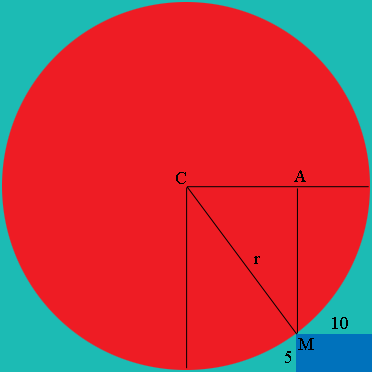SEARCH HOMEMath Central Quandaries & QueriesQuestion from mazhar, a student: suppose the length and breadth of the rectangle are 5 cm and 10 cm respectively and M is a point along the corner of the circle. what is the radius of the circle?(diagram is given..but i didn't mention it ..actually the diagram looks like a circle inscribed in a square and the right bottom corner one rectangle will be given ,it is touches to circle at a point M that i've already mentioned and the dimensions of that rectangle also i've mentioned) please help me out..Mazhar,

I drew a diagram of what you described, added some lines and labeled some points.Triangle $ACM$ is a right triangle. Whenever I see a right triangle I ask myself "What does Pythagoras Theorem tell me?"

Penny* Registered trade mark of Imperial Oil Limited. Used under license.Math Central is supported by the University of Regina and the Imperial Oil Foundation.